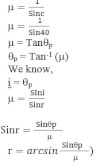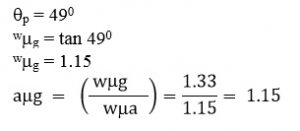# Brewster’s Law

Brewster’s law states that the tangent of angle of polarisation is numerically equal to the refractive index of transparent medium. If qp be the angle of polarization and µ be the refractive index of transparent medium.
then
tanθp = μ

Explain light is transverse wave:

Critical angle for a certain wavelength of light in glass is 40 calculate the polarized angle and angle of refracting in glass corresponding to this.
We know,A beam of light travelling in water strikes an angle plate which is also immersed in water. When the angle of incidence is 49. The reflected beam is found to be polarized what is the refractive index of glass.
Here,
For water glass interface,